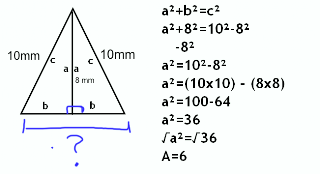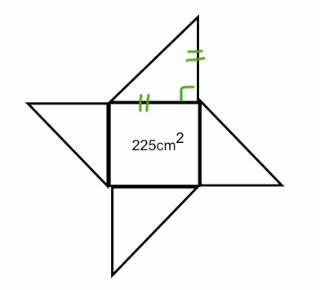### Pythagaros

Thursday, February 26, 2009TRIANGLE

So this is a right triangle also known as R.A.T. It is called a right triangle because it has that little square that tells you it's 90°. Well the 90° is made by two parts of the triangle, that is θ (theta) and B (beta). Those two angles make 90° are called complementary angle. Hypotenuse is the longest side which is often called or labeled as "C". Just remember that is it across from the right angle. The two smaller sides are often labeled as "A" or "B", it doesn't matter which side it is on but now across the right angle.

This is a special type of rectangle. A square has 4 equal sides. You can tell that it has 4 equal sides because it has those lines on the sides(lines of symmetry). A square is made up of four 90° angles, one at each corner. The whole square makes 360° because of the four 90° angles. In this square, there are 2 right angle triangles. You know this because if you cut a line from one corner to the opposite corner and it'll make the 2 R.A.Ts.

PYTHAGOREAN THEOREM
This is the Pythagorean Theorem. The length of a side of a right angle triangle. Well what the theorem means is that, if you know side "a" and side "b", then all you do is square them. From there, you will need to add them together, which will leave you with side "c" squared. Find the square root of the answer and that will give you the length of side "c".

PYTHAGORAS
-father of math
-hung out in Egypt
-harmonics
-vegan
-cosmos
-circumference of the Earth
-universe Earth around the sun

VOCABULARY
Legs: make the right angle. Legs of R.A.T. are (a) and (b).
Hypotenuse: is the longest side of a triangle.
R.A.T: this stands for Right Angle Triangle
Greek: is a person who lives or born in Greece.
Theorem: a formula or a theory that can be proven

Problem #1Problem #2You need to find out how long each side of the square is. Then that will give you side "A" and "B" for the triangles since they're both the same length. Ok so, like a normal question find the length of the hypotenuse, which is 'c'. Once you have got that you can now label the triangle and add up all the sides to find the perimeter.

These are my videos with Carrie and Arielle.